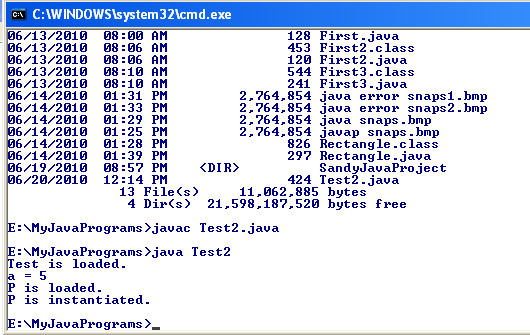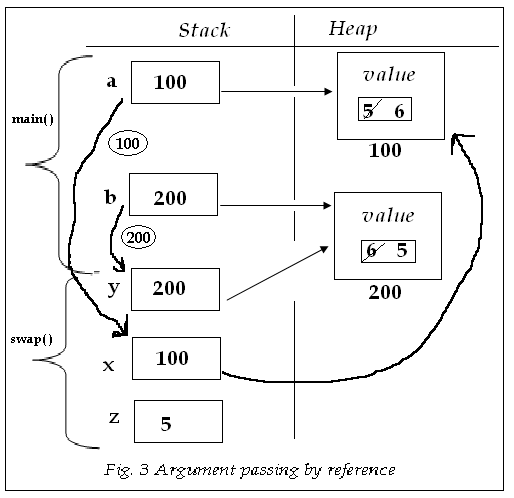• www.uptuexam.com
• GBTU Results 2014
• MTU Results 2014
• UPTU Circulars
• COP Result 2012,13,14
• UPTU Previous Papers
• GATE study material
• Robotics Projects
Thank you for visiting www.UPTUexam.com " Free download Exam materials, previous year question papers, free ebooks & Providing Important Questions To Do their Exams Well and Totally a FREE SERVICE for all engineering streams (B.Tech)."

### Limitations of static methods & static block

##### Limitations of static methods & static block:-

o   Only static data members of a class can be referred in a static block or method.
o   A static block or static method can directly invoke only static methods.

o   ‘this’ and ‘super’ keyword cannot be used in a static method or in  a static block.

Program below executes without ‘main’ method –
class Test
{
static
{
System.out.println(“It is executing without main…..”);
System.exit(0);
}
}

Not only we can do this much but also we can do list of things. See the program below : -

1. class Test2
2. {
3. int a;
4. public Test2(int x)
5. {
6. a = x;
7. }

8. public void display1()
9. {
10. System.out.println("a = " + a);
11. }

12. static
13. {
15. Test2 t = new Test2(5);
16. t.display1();
17. P x = new P();
18. System.exit(0);
19. }
20. }

21. class P
22. {
23. public P()
24. {
25. System.out.println("P is instantiated.");
26. }

27. static
28. {
30. }
31. }

OUTPUT -Now, as we saw that we can do almost everything without having main( ), then why we need main( ) in our program?

Passing arguments to methods-

In Java, primitive type arguments are passed to methods by value i.e. their copy is created in the invoked method.
Objects are passed by references i.e. in case of objects, copy of their reference variables is created in the invoked method.

Now the program below is written to swap the values.

Observe it carefully to understand how this issue is being resolved.

1. public class Swapper
2. {
3. public static void swap(int x, int y)
4. {
5. int z;
6. z = x;
7. x = y;
8. y = z;
9. }
10. }
11. public class SwapperTest
12. {
13. public static void main(String[ ] args)
14. {
15. int a =5, b = 6;
16. System.out.println("a = " + a);
17. System.out.println("b = " + b);
18. Swapper.swap(a, b);
19. System.out.println("After swap");
20. System.out.println("a = " + a);
21. System.out.println("b = " + b);
22. }
23. }

The output is  -Our purpose is not solved. Let’s see the reason for this.

See the fig2. belowNow, let’s find out solution to this problem.

1. public class MyNumber
2. {
3. int value;
4. public MyNumber(int x)
5. {
6. value = x;
7. }
8. }
9. public class Swapper
10. {
11. public static void swap(MyNumber x, MyNumber y)
12. {
13. int z;
14. z = x.value;
15. x.value = y.value;
16. y.value = z;
17. }
18. }
19. public class SwapperTest
20. {
21. public static void main(String[ ] args)
22. {
23. MyNumber a = new MyNumber(5);
24. MyNumber b = new MyNumber(6);
25. System.out.println("a = " + a.value);
26. System.out.println("b = " + b.value);
27. Swapper.swap(a, b);
28. System.out.println("After swap");
29. System.out.println("a = " + a.value);
30. System.out.println("b = " + b.value);
31. }
32. }

Output –Now the problem is fixed as you can see the value is swapped. We handled it by passing value by reference.
Let see the explanation diagrammatically. (Fig. - 3)Question. Define a class named Rational that contains 2 data members to store the value of numerator and denominator of a rational number, default & two parameterized constructors, a display ( ) method that displays the value of a rational object in    form and add ( ) methods which are referenced by the following class.

1. package StaticConceptualPrograms;
2. public class Rational
{
int numerator, denominator;

public Rational() { }

public Rational(int x, int y)
{
numerator = x;
denominator = y;
}

void display()
{
System.out.println(numerator+"/"+denominator);
}

{
numerator = y.denominator*this.numerator + this.denominator * y.numerator;

denominator = y.denominator*this.denominator;

return this;
}

{
numerator = y.denominator*x.numerator + x.denominator * y.numerator;

denominator = y.denominator*x.denominator;

return this;
}

/*
static Rational add(Rational x, Rational y)
{

}
*/
}

package StaticConceptualPrograms;

public class RationalTest
{
public static void main(String[] args)
{
Rational a = new Rational(2, 3);
Rational b = new Rational(4, 5);
System.out.print("Rational a is : ");
a.display();
System.out.println("");

System.out.print("Rational b is : ");
b.display();
System.out.println("");

3. System.out.print("Sum of a & b : ");
c.display();
System.out.println("");

Rational r = new Rational(6, 5);
Rational s = new Rational(4, 3);
Rational t = new Rational();

System.out.print("Rational r is : ");
r.display();
System.out.println("");

System.out.print("Rational s is : ");
s.display();
System.out.println("");

System.out.print("Rational r & s is : ");
t.display();
System.out.println("");

Rational z;
System.out.print("Sum of a, b, r & s is : ");
//z.display();
}
4. }

Output -1. public class Rational
{
int p, q;

public Rational() {}
2. public Rational(int x, int y)
{
p = x;
q = y;
}

public void display()
{
System.out.println(p+"/"+q);
}

{
Rational s = new Rational();
s.p = p * r.q + q * r.p;
s.q = q * r.q;
return s;
}

public void add(Rational a, Rational b)
{
p = a.p * b.q + a.q * b.q;
q = a.q * b.q;
}

public static Rational add(Rational a, Rational b)
{
Rational c = new Rational();
c.p = a.p * b.q + a.q * b.q;
c.q = a.q * b.q;
return c;
}
}

public class RationalTest
{
public static void main(String[] args)
{
Rational a = new Rational(2, 3);
Rational b = new Rational(4, 5);
System.out.print("Rational a is : ");
a.display();
System.out.println("");

System.out.print("Rational b is : ");
b.display();
System.out.println("");

System.out.print("Sum of a & b : ");
c.display();
System.out.println("");

Rational r = new Rational(6, 5);
Rational s = new Rational(4, 3);
Rational t = new Rational();

3. System.out.print("Rational r is : ");
r.display();
System.out.println("");

System.out.print("Rational s is : ");
s.display();
System.out.println("");

System.out.print("Rational r & s is : ");
t.display();
System.out.println("");

Rational z;
System.out.print("Sum of a, b, r & s is : ");
z.display();
}
}

To understand the logic used inside the different methods of Rational class, please see the diagrams belowFigure (20.a) to understand public Rational add(Rational r) methodProgram -

1. class Test1
{
int a, b;

public Test1(int a, int b)
{
a=a;
b=b;
}
public static void main(String[] args)
{
Test1 t = new Test1(5, 6);
t.display();
}

public void display()
{
System.out.println("a = " + a);
System.out.println("b = " + b);
2.                }
}

Output -